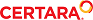﻿ Example - Model circadian rhythm, inter-occasion variability, and two epsilons

Modeling circadian rhythm, inter-occasion variability, and two epsilons

This example is based on the study of the pharmacokinetics of midazolam reported by Van Rongen, A., et al. (2015) (“Population Pharmacokinetic Model Characterizing 24-Hour Variation in the Pharma­cokinetics of Oral and Intravenous Midazolam in Healthy Volunteers”. CPT: Pharmacometrics & Sys­tem Pharmacology, 4.8:454–464). The aim of this study was to evaluate 24-hour variation in the pharmacokinetics of the CYP3A substrate midazolam.

This example shows an implementation of the cosinor procedure for describing 24 hour variation. This project also illustrates the visual predictive check results with stratification on inter-occasion variables and how to implement a two dosepoint model with two epsilons, one for oral and the other one for IV using Textual Mode.

Open the project

Two datasets are listed in the Data folder. The dataset midazolam_sim consists of observations CObs, covariate OCC, which depends on the time of dosing and includes 6 dosing occasions (OCC=1 (10:00 AM) 2 (02:00 PM), 3 (06:00 PM), 4 (10:00 PM), 5 (02:00 AM), 6 (06:00 AM)), covari­ate OCCTime, representing the time of dosing in minutes starting at midnight, where TAD is time after dosing in minutes and is mapped to Time to avoid unnecessary computations. There are 12 sub­jects for each dosing period.

The input doses in the dataset midazolam_dose are the same as in the mentioned article. The PO dose separated in time from IV bolus by 150 minutes.

Review the model text

1. In the Object Browser, expand the Workflow and select the MidazolamModel object.

2. Select Model in the Setup list. The Model text panel is displayed.

The value of bioavailability (F1) oscillates around mesor, and is defined in the structure model param­eters as follows:

F1=tvF1*exp(nF1+IOV)+ tvAMF*cos((6.283/tvFreq)*(t+OCCTime – tvACF))

where:

tvF1 presents the population mean of bioavailability (theta)
nF1 is the Inter-individual variability (eta)
IOV is the interoccasion variability implemented in the following statement with inter-individual variability incorporated inside.

IOV=(OCC==1)*nF1_OCC1+(OCC==2)*nF1_OCC2+(OCC==3)*nF1_OCC3+(OCC==4)*nF1_OCC4+(OCC==5)*nF1_OCC5+(OCC==6)*nF1_OCC6

tvAMF is the amplitude of cosine function
tvFreq is the period of cosine function (24 hours)
tvACF is the acrophase (time to peak of the cosine function)
t+OCCTime represents the time in minutes starting at midnight.

The clearance is defined similarly as the bioavailability. For the absorption rate constant Ka, a multi­plication factor at 2:00 PM is added by applying of the ternary operator:

Ka=(OCC == 2 ? tvKa*exp(nKa)*tvKaMult : tvKa*exp(nKa))

It is worth noting that the multiplication factor in the absorption rate was found in Van Rongen, A., et al. (2015) to give a better fit to the clinical data.

Note that for this example the value of the standard deviation for the residual error changes after TAD reaches 150 minutes; that is, there are two epsilons (epsilon 1: oral, epsilon 2: IV bolus 150 minutes after oral). To incorporate this, a fixed effect is introduced using the following statements:

bIV=(t >= 150 ? 1 : 0)
error(CEps= 0.163723)
observe(CObs=Central*(1+(1+bIV*Coeff_IV)*CEps))

The above statements means that if TAD is less than 150 minutes, the standard deviation for the residual error is 0.163723. Otherwise, it is (1+Coeff_IV)* 0.163723.

Execution of VPC model

1. In the Object Browser select VPC MidazolamModel object.

This model is a copy of the previous one with the values for the fixed and random effects chosen as the final estimated parameter values.

2. Select the Run Options tab.

3. Make sure that Predictive Check run mode is selected.

4. In the Main sub-tab, leave the categorical covariate OCC selected in the Stratify menu.

5.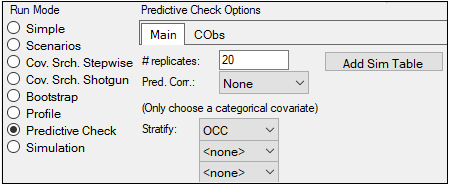6. Switch to the CObs sub-tab and make sure that the Quantile checkbox is checked.

7. Click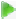(Execute icon).

8. Select Pop PredCheck ObsQ_SimQ in the Plots part of the Results tab.

For each strata, the observed quantiles are superimposed with predictive check quantiles over the observed data. The results are shown in the image below, which shows a good match between observed and predicted data.

9.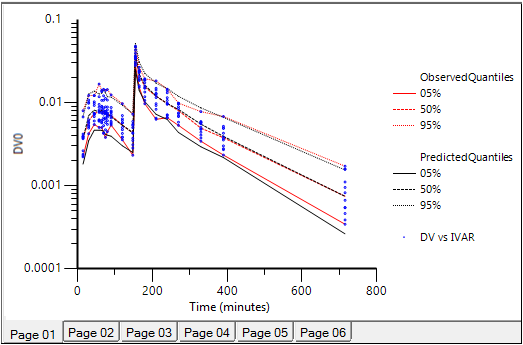Predictive Check plot for VPC MidazolamModel. Red lines: observed quantiles, black lines: pre­dicted quantiles, blue symbols: observed data.

10. Select Pop PredCheck_ObsQ_SimQCI in the Plots part of the Results tab.

PredCheck_ObsQ_SimQCI plot of confidence intervals of the simulated quantiles is generated due to checked Quantile checkbox in the Predictive Check Options area: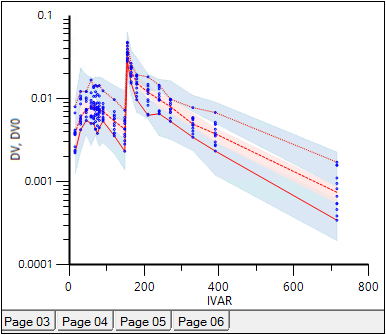Predictive Check plot with confidence intervals of the simulated quantiles for VPC Midazolam­Model.

In each plot, the two blue shaded areas respectively represent the confidence intervals for the simu­lated 5th and 95th quantiles, and the red shaded area represents the confidence interval for the 50th quantile. The red lines inside these shaded areas denote the median of these simulated quantiles.

In this project, the lines that define the outer edge of the shaded areas have been removed by double-clicking on the plot and setting Line Style to None for each of the Predicted Quantiles graphs that named “05%, 05%” through “95%. 95%”.